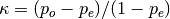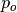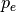# sklearn.metrics.cohen_kappa_score¶

sklearn.metrics.cohen_kappa_score(y1, y2, labels=None, weights=None)[source]

Cohen’s kappa: a statistic that measures inter-annotator agreement.

This function computes Cohen’s kappa [R199], a score that expresses the level of agreement between two annotators on a classification problem. It is defined aswhereis the empirical probability of agreement on the label assigned to any sample (the observed agreement ratio), andis the expected agreement when both annotators assign labels randomly.is estimated using a per-annotator empirical prior over the class labels [R200].

Read more in the User Guide.

Parameters: y1 : array, shape = [n_samples] Labels assigned by the first annotator. y2 : array, shape = [n_samples] Labels assigned by the second annotator. The kappa statistic is symmetric, so swapping y1 and y2 doesn’t change the value. labels : array, shape = [n_classes], optional List of labels to index the matrix. This may be used to select a subset of labels. If None, all labels that appear at least once in y1 or y2 are used. weights : str, optional List of weighting type to calculate the score. None means no weighted; “linear” means linear weighted; “quadratic” means quadratic weighted. kappa : float The kappa statistic, which is a number between -1 and 1. The maximum value means complete agreement; zero or lower means chance agreement.

References

 [R199] (1, 2) J. Cohen (1960). “A coefficient of agreement for nominal scales”. Educational and Psychological Measurement 20(1):37-46. doi:10.1177/001316446002000104.